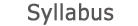2018 - 2019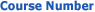0512-2525-01
Electromagnetic Fields
FACULTY OF ENGINEERING | ELECTRICAL ENGINEERING AND ELECTRONIC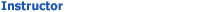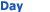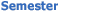Prof. Steinberg Benny 020 Mon 1200-1500 Sem  1
University credit hours:  4.0

Course description
Credit Points: 3.5
Prerequisites: Harmonic Analysis; Physics (2); Partial Differential Equations (concurrent)

Electromagnetic forces and fields and their sources. Maxwell’s equation in vacuum: The integral and differential forms; boundary and continuity conditions; static and dynamic problems; plane waves; the quasistatic field equations.Electro-quasistatics: Potential; Poisson’s and Laplace’s equations; the superposition integrals; boundary value problems in Cartesian, cylindrical and spherical coordinates; electro-mechanical systems and equivalent circuits; numerical solutions. Magneto-quasistatics: The vector potential and gauge theory; Biot-Savart superposition integral; boundary value problems; induced fields and potentials; self and mutual induction; magneto-mechanical systems and equivalent circuits. Fields in matter: Conduction and charge relaxation, polarization and magnetization; physical models; field equations and constitutive relations; solution techniques; examples for quasistatic electro- and magneto-mechanical systems. Electromagnetic energy: Pointing's theorem; energy balance in static and quasistatic problems; exchange of mechanical and electromagnetic energy. Electro-dynamics: The sinusoidal steady state and introduction to electromagnetic waves.

accessibility declaration